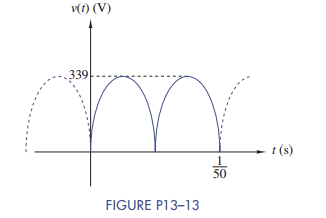### Create an Account

Home / Questions / Use the results in Figure 13 4 to calculate the Fourier coefficients of the full-wave rec...

# Use the results in Figure 13 4 to calculate the Fourier coefficients of the full-wave rectified sine wave in Figure P13–13 Use MATLAB to verify your results Write an expression for the

Use the results in Figure 13–4 to calculate the Fourier coefficients of the full-wave rectified sine wave in Figure P13–13. Use MATLAB to verify your results. Write an expression for the first four nonzero terms in the Fourier series.May 24 2020 View more View LessSubscribe To Get Solution